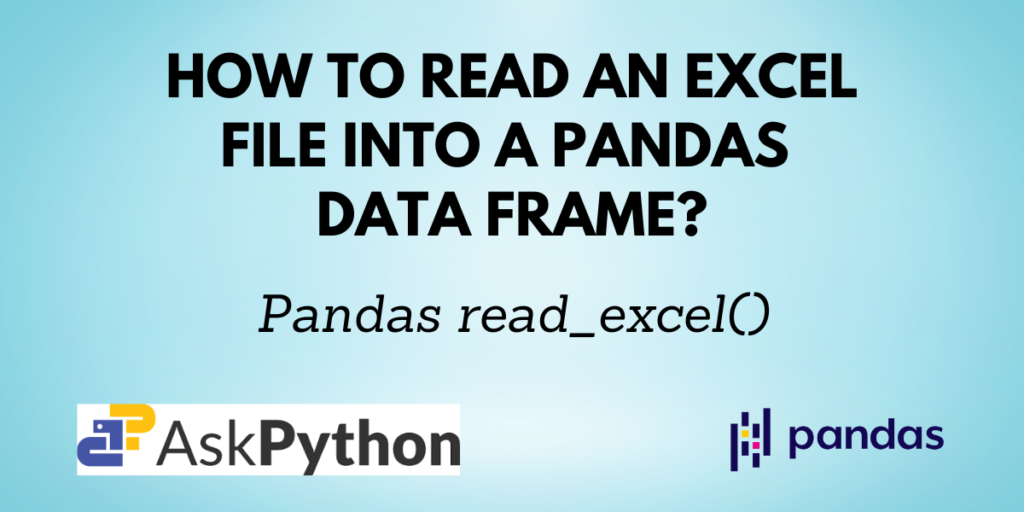Microsoft Excel is software that enables users to manage, format, and organize data in the form of a spreadsheet. The data is presented as 2-dimensional tables in an Excel file. Pandas also have a data structure similar to tables, a data frame.

You have previously learned to read data from CSV, JSON, and HTML format files. In this tutorial, you will understand how you can read an Excel file into a Pandas DataFrame object by using the `pandas.read_excel()` method.

You need to have Python and Pandas installed on your computer and your favorite IDE set up to start coding. If you don’t have Pandas installed, you can install it using the command:

```pip install pandas
```

If you are using the Anaconda distribution use the command:

```conda install pandas
```

```pandas.read_excel(io,
sheet_name=0,
*,
names=None,
index_col=None,
usecols=None,
squeeze=None,
dtype=None,
engine=None,
converters=None,
true_values=None,
false_values=None,
skiprows=None,
nrows=None,
na_values=None,
keep_default_na=True,
na_filter=True,
verbose=False,
parse_dates=False,
date_parser=None,
thousands=None,
decimal='.',
comment=None,
skipfooter=0,
convert_float=None,
mangle_dupe_cols=True,
storage_options=None)
```

Some of the important parameters are:

## Reading an Excel file using Pandas

Let us first have a look at the sample Excel sheets. The name of the Excel file here is ‘info.xlsx‘. It contains two sheets as shown below:

To read the Excel file, use the below code:

```import pandas as pd

df
```

Since the data is in the form of a Pandas DataFrame now, you can operate it just like you operate any other DataFrame.
For example, if you want to get only the column names, you can write:

```print(df['car'].to_list())
```

Output:

```['ciaz', 'swift', 'i20', 'kia', 'brezza']
```

## Specifying sheet names

By default, only the first sheet in the Excel workbook or file is read by the `read_excel()` function. To read multiple sheets from an Excel file, use the sheet_names parameter. You can mention an integer or a list of integers that represent the 0-indexed sheet number sequence to be read.

Since the above workbook has two sheets, the car has an index of 0 and the flower has an index of 1.

```df = pd.read_excel('info.xlsx', sheet_name = 0)
df
```
```df = pd.read_excel('info.xlsx', sheet_name = 1)
df
```

## Displaying only some specific columns in the output

If you don’t want all the columns in the Excel sheet, you can select the columns that you need using the usecols parameter.

```df = pd.read_excel('info.xlsx', sheet_name = 0, usecols=['car', 'price'])
df
```

As the code suggests, only the columns car and price from sheet 0 i.e. car are displayed and other columns are not included in the resulting DataFrame.

## Changing column names

You can also change the column names for the Excel sheet, if needed, using the names parameter in the `read_excel()` function.

```df = pd.read_excel('info.xlsx', sheet_name = 1, names=['col 1', 'col 2'])
df
```

The names of the columns from sheet 1 i.e. flower have been changed to col 1 and col 2.

## Skipping rows

If you have any irrelevant or redundant data and do not want it in your DataFrame object, you can assign an integer or a list of integers to the skiprows parameter. If an integer n is given, it skips the first n rows, and if a list of 0-indexed integers is given, those rows are only skipped.

```df = pd.read_excel('info.xlsx', sheet_name=1, skiprows=1)
df
```

One row from the flower sheet is skipped here.

When you use the header parameter with the `read_excel()` function, you assign an integer n to it and then the function treats the nth row as the header and displays only the data below it. The rows above it are discarded.

```df = pd.read_excel('info.xlsx', sheet_name = 0, header = 3)
df
```

The third row from the car sheet is set as the header, and the two entries above it are discarded. Only the rows below it are displayed.

## Selecting only ‘n’ rows

The nrows parameter lets you choose how many rows you want in your resulting Pandas DataFrame. It displays the first n rows, where n is the value assigned to the parameter.

```df = pd.read_excel('info.xlsx', nrows=3)
df
```

## Conclusion

The `pandas.read_excel()` function lets you read any Excel file into a Pandas DataFrame object. It also provides various parameters which you can use to customize the output as per your requirements, some of which were discussed in this tutorial.